# "if" .... "and"..... "or" ..... "while" ...

## Recommended PostsI am currently working on a LISP that will draw a plan of a piping elbow.

Before the draughting program runs, I need the LISP to analyse the angle entered by the user to determine whether it is equal or not to 90 or 180 degrees.

If the angle is not equal to either of these, then the program needs to run as planned.

I have tried using something like this....

```(if
(or (\= elbang 90) (\= elbang 180))
(progn
"run elbow draw program"
) ; end progn
) ; end if
```

however I really dont understand how the "OR", "AND", "WHILE" & "NOT" functions work.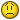##### Share on other sitesOR: if THIS or THAT, then do something (just has to be one or the other)

AND: if THIS & THAT, then do something (has to be both)

WHILE: while something (= (getvar "cmdecho" 0)), then do something

NOT: if something is not something, do something

(if (not (equal (getvar "clayer") 0)

);not

(setvar "clayer" 0)

);if

##### Share on other sitesThanks for the information Alanjt, much appreciated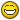; but can you see any error in my posted code regarding the use of "or" (apart from the obvious no "defun" etc)?

##### Share on other sitesThanks for the information Alanjt, much appreciated; but can you see any error in my posted code regarding the use of "or" (apart from the obvious no "defun" etc)?

try this:

```(if
(or ([color=Red]/[/color]= elbang 90) ([color=Red]/[/color]= elbang 180))
(progn
"run elbow draw program"
) ; end progn
) ; end if```

##### Share on other sites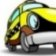Lee Mac

There are many ways to approach this problem but before a solution you need to

clarify the process. In your example you are using integers 90 & 180. To me this implies

that they are user entered values because any angle you get from an entity like a line

will be a real number & likely be in radians not degrees.

So which is it?

Note that often a fuzz factor must be used as well for values that are near the desired value.

This is especially true with ACAD as there are rounding errors.

This is your example corrected & doesn't give the correct result, you used the wrong SLASH character

```(if (or (/= elbang 90) (/= elbang 180))
(progn
"run elbow draw program"
)
) ; end if```

In the simple example you gave I would do this, assuming that only integers would be tested.

```;;  elbang is /= 90 AND /= 180
(if (and (/= elbang 90) (/= elbang 180))
(progn
(princ "run elbow draw program")
)
) ; end if```

```;;  OR returns true if either is true
;;  OR returns nil if Both are false
;;  so if Both are false, NOT returns True
(if (not (or (= elbang 90) (= elbang 180)))
(progn
(princ "run elbow draw program")
)
) ; end if```

```;; Another way to test
;;  if var is not a member of the list
(if (not (member elbang '(90 180)))
(progn
(princ "run elbow draw program")
)
) ; end if```

```;; Another way to test
;;  if var is not a member of the list
(if (not (vl-position elbang '(90 180)))
(progn
(princ "run elbow draw program")
)
) ; end if```

```;; This one is a little tricky
;;  returns True if both test are true, like this
;;  (setq elbang 20)
;;  (/= 90 elbang) -> true
;;  (/= elbang 180) -> true
;;  both true then the prg will execute
;;  (setq elbang 90)
;;  (/= 90 elbang) -> flase
;;  (/= elbang 180) -> true
;;  any false will return false then the prg will NOT execute
;;  This is like an AND,
(if (/= 90 elbang 180) ; elbang must be in the middle
(progn
(princ "run elbow draw program")
)
) ; end if```

##### Share on other sitesThanks for the information guys, (CAB, you really went to town with that last post - very informative, thanks).

I now understand the functions a lot better.I realised my mistake with the posted LISP, but thanks for taking the time to point it out.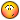To better clarify my intentions -

An initial "User Input program" is run to gain user values - including

• Equipment Type

• View (Plan/Elevation)

• Point for Insertion

• Pipe Nominal Bore (50/80/100/...etc)

• (For Elbow) - Radius (Long/Short/5D)

• (For Elbow) - Angle

Depending upon which Equipment type is entered, various other programs will run and construct the selected equipment to the view and dimensions selected.

To resolve my issue with the use of the "
and/or
" command, I have qualified each scenario and used an "
if
" function to separate each.

i.e.

```(if (and (/= elbang 90.0) (< elbang 180.0))
(progn
"draw elbow"
) ; end progn
) ; end if

(if (= elbang 90.0)
(progn
"draw 90.0 elbow"
) ; end progn
) ; end if

etc etc```

This seems to work well.Once again, thanks for all your help.##### Share on other sitesGlad I could shed some light on the subject.

When you have several tests and only one will be true or you expect one will be true if tested in

a specific order then the COND is the function to use. See this example.

```(cond
((equal elbang 90.0 0.0001) ; this will catch rounding errors
(princ "draw 90.0 elbow") ; go do it
)
((< 90.0 elbang 180.0) ; it is between 90 and 180
(princ "draw elbow")
)
(t ; elbang is < 90 OR >= 180
;; Do what ever
)
)```

##### Share on other sitesI suppose the "cond" function is tidier than the "if" function - although I find the "if" function can still produce the desired results. I'm not aware of any drawbacks of using such a function for this purpose.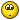There is one more question I would like to ask - its been on my mind for a while now...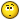What exactly does the "progn" prefix mean/do?

At first, I thought that the "progn" prefix was used specifically with "if" functions to inform the program that there will be more than one line of programming before the "if" command decides what to do with the data. However, in a previous thread, I notice that you have used the "progn" prefix with a "while" function...

##### Share on other sitesWell the COND is the right tool for the job. Just a matter of choice.

PROGN is a wrapper for a group of code.

The WHILE

Evaluates a test expression, and if it is not nil, evaluates other expressions;

repeats this process until the test expression evaluates to nil

The PROGN makes the entire group the TEST expression.

Other ways to use the in a WHILE loop is to use COND or AND

If you wrap a group of code in an AND each line will execute until a nil occurs. At that point the

AND exits with a nil returned to the WHILE which exits the loop.

The COND can be used the same way.

##### Share on other sitesLISP Logic and More by Charles Alan Butler aka CAB

Here is my take on the subject of LISP Logic functions & there usage.

Explore with me the if and or cond while & progn functions.

The if statement works like this

```(if (this is true)
(then do this)
)```

```(if (this is true)
(then do this)
(else do this when false)
)```

The (cond) stmt on the other hand executes the all of code following

a true condition and then exits the condition stmt. It returns value of the last

expression in the sublist. If there is only one expression in the sublist (that is,

if result is missing), the value of the test expression is returned.

```(cond
((= 1 0)
none of this will get executed
because the conditions is false
) ; end cond 1

((= 1 1)
do all of this because it is true
and this
and this
) ; end cond 2

((= 2 2)
none of this will get
executed even if it is true
because the prior stmt was true
) ; end cond 3

(T
This is often placed at the last position
in a condition statement and will be executed
if all the prior conditions are false
if any one of the above conditions are true
this will not be executed
) ; end cond 4
) ; end cond stmt```

More on the condition statement in a minute.

The OR will process each line as long as they are false, it will quit when true is returned

```(or (a. is true) ; stop here
(b. does not get this far)
)```

```(or (a. is false) ; keep going
(b. do this & if false keep going)
(c. do this if b was false)
)```

The AND will process each line as long as they are true, it will quit when false is returned

```(and (a. is true) ; keep going
(b. do this & if true keep going)
(c. do this if b was true)
)```

Note that AND & OR themselves only return true or nil,

whereas IF and COND will return the value of the expression.

```(setq rtn
(or (+ 3 7) ; returns 10 which is not nil so it passes the test for true
(+ 2 4 6) ; return 12
)
)```

While the value in rtn is t

```(setq rtn
(if (+ 3 7) ; returns 10 which is not nil so it passes the test for true
(+ 2 4 6) ; return 12
)
)```

The value in rtn is 12

```(setq rtn
(if (> 3 7) ; 3 is not > 7 so this returns nil
(+ 2 4 6) ; return 12
)
)```

The value in rtn is nil because there was no else line to consider

```(setq rtn
(if (> 3 7) ; 3 is not > 7 so this returns nil
(+ 2 4 6)
(- 10 2)
)
)```

No supprise here, the rtn value is 8

The cond statement works simular to the if

Here is an example I like

```(initget 0 "Yes No")
(setq ans
(cond
("Yes")
)
)```

If the user hits the enter key nil is returned and the cond returns "Yes"

If the user enters text the text is returned

Some examples of (progn

Note that progn returns the value of the LAST evaluated expression.

```(if (this is true)
(progn
do all of this
and this
and this
)
(don't do this)
); endif

(if (this is false)
(don't do this)
(progn
do all of this
and this
and this
)
)```

So you see the progn is a way to group code together.

The (if) stmt will execute the group of code when true and

will execute the second group of code when false,

if there is a second group of code. If you forget the (progn) only the first

line of code will be executed if true and the rest skipped.

There are other ways to group code together and you will learn them in time.

The (cond) stmt on the other hand executes all of the code following

a true condition and then exits the condition stmt. Remember true is anything not nil.

```(cond
((= 1 0)
none of this will get executed
because the conditions is false
) ; end cond 1

((= 1 1)
do all of this because it is true
and this
and this
) ; end cond 2

((= 2 2)
none of this will get
executed even if it is true
because the prior stmt was true
) ; end cond 3

(T
This is often placed at the last position
in a condition statement and will be executed
if all the prior conditions are false
if any one of the above conditions are true
this will not be executed
) ; end cond 4
) ; end cond stmt```

Here is a trick for the cond statement when you want to conditionally execute the cond.

Often we see it like this:

```(if (something is true) ; reminder, 'true' here is really anything (not nil)
(cond
((= 1 1) ...)
((= 2 2) ...)
) ; end cond stmt
) ; endif```

It can be written like this so that if something is not true the first condition is true &

nothing is done but the cond statement is satisfied that it found a true condition.

The processing skips the remaining conditions.

```(cond
((not (something is true))) ; if something is not true stop here
((= 1 1) ...)
((= 2 2) ...)
) ; end cond stmt```

The WHILE function as a conditional loop and continues the loop until the next

expression returns nil. Here we test the value in cnt looking for it to become 0

so that we can exit the while loop. The code within the loop is executed 5 times,

while the cnt value is 0 through 4.

```(setq cnt 0)
(while (= cnt 5)
;;  do some stuff
(setq cnt (1+ cnt))
)```

It can also be written like this and the cnt value is 0 through 4.

```(setq cnt -1)
(while (= (setq cnt (1+ cnt)) 5)
;;  do some stuff
)```

Sometimes the condition you want to test is set at the end of the code within

the loop. The code may look like this.

```(setq continue_loop t)
(while continue_loop
;;  do some stuff
(if (I want to leave the loop is true)
(setq continue_loop nil) ; flag to exit the loop
)
)```

This can also be written like this:

```(setq continue_loop t)
(while
(progn
;;  do some stuff
(not (I want to leave the loop is true)) ; flag to exit the loop
) ; progn
)```

So you see that when (I want to leave the loop is true) returns true, the not will

return nil and because it is the last expression in the progn, that is the value the

while sees. It then exits the loop.

Here is a real world example.

This is my solution for entering numbers like key words

The simulated key words would be "90 180 270 -90"

```(while
(progn
(setq rang
(cond ((getint "\nEnter the rotation angle [90/180/270] <90>"))
(90)))
(if (not (vl-position rang '(90 180 270 -90)))
(not (prompt "\nError  Angle must be 90 180 270, please re-enter.")))
)
)```

That's all for now.

See ya.

##### Share on other sitesThat tutorial was absolutely fantastic Charles, Thanks. Until now, I was following an AutoLISP reference manual for R11, and it only described the if, or, while, not, and cond functions in very brief detail.

I now understand them a lot better.

So, if I have understood it correctly, a cond stmt doesnt need a wrapper like progn, whereas the if and while stmts sometimes do.

Also, you can combine a cond with the initget and getkword to get a "default" when obtaining a keyword from a user.

Once again, thanks for all your help and teachings - both are much appreciated.##### Share on other sitesThe Help file for lisp is still very brief.

The Progn is another tool. As your knowledge grows you will find more & more uses for it.

Same with COND. Maybe I have another example here. I'll look.

##### Share on other sitesHere is an example of WHILE with code wraped with a AND wraped with a NOT

;; Sel by Type.lsp

```;;  CAB 11.06.2007
;;  Get a selection set of thawed objects in current space with matching type
;;  Option to match layer too
;;  Highlights the selected items & returns the selection set
(defun c:oSel (/ fuzz ent obj layFilter Area2match lst ss ss2)
(setq fuzz  0.001)
(while
(not
(and
(or (setq ent (car (entsel "\nSelect object to match object type & layer.")))
(prompt "\nMissed, try again."))
(setq obj (vlax-ename->vla-object ent)
typ (cdr(assoc 0 (entget ent))))
(or (not (vl-position typ '(""))) ; Add type names here ("DIMENSION" "LEADER")
(prompt "\nObject type not supported, try again."))
)
)
)
(initget "Yes No")
(if (equal (getkword "\nMatch layer too? [Yes/No]<Yes>: ") "No")
(setq layFilter '(8 . "*"))
(setq layFilter (cons 8 (vla-get-layer obj)))
)
(prompt "\nSelect objects or ENTER for All objects in this space.")

(or (setq ss (ssget (list layFilter (cons 0 typ)(cons 410 (getvar "ctab")))))
(setq ss (ssget "_All" (list layFilter (cons 0 typ)(cons 410 (getvar "ctab"))))))
(sssetfirst)
(print)
(if (and ss (not(zerop(sslength ss))))
)
)```

## Join the conversation

You can post now and register later. If you have an account, sign in now to post with your account.
Note: Your post will require moderator approval before it will be visible.×   Pasted as rich text.   Paste as plain text instead

Only 75 emoji are allowed.